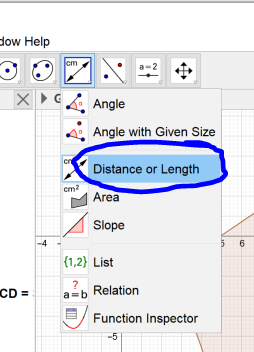# Measure Tool

MrLadas shared this problem 2 years ago
Not a Problem

I am an elementary teacher trying to use the measure tool with my students to create ratios of perimeter to diameter of regular polygons. When we measure the perimeter of a regular polygon, and divide it by the diameter (based off of the values from the 'measure' tool), we do not get consistent answers. Are we using the measure tool incorrectly?

Thank you,

Peter1

Bonsoir,

que désigne le "diameter" ?

le plus simple serait de poster un fichier1

Pour moi, pour n >=4 et n pair, cela peut s'illustrer ainsi1

Merci,

I hope my example makes sense.1

yes1

```Perimeter(poly1) / DB
Perimeter(poly1_{1}) / distanceD1B1
Perimeter(poly1_{2}) / distanceD2B2```

all give the same for me1

I did not record this way. Thank you. I am new using this GeoGebra. I did it by calculator - I figured out the problem ... I am using the rounded values, which is inaccurate. How do you use the calculator on GeoGebra to be more accurate?

Thank you so much.1

You can also type in what I've put above1

Michael,

You are kind to be so helpful. Thank you. I found the digits setting for rounding. The only other thing is your 'Perimeter...' formulae. Is that just typed in manually, or is there a tool to create these calcuations?

Peter1

You can type it or use the tool: